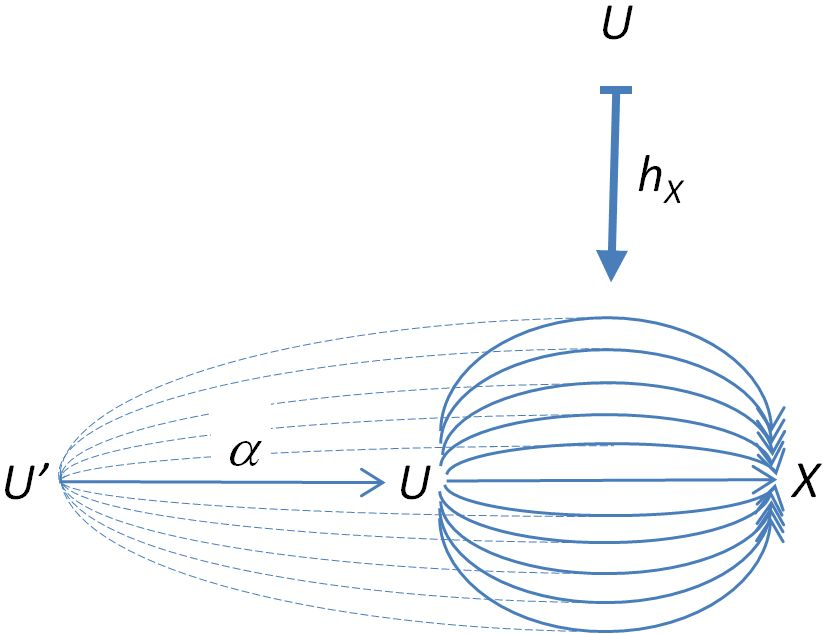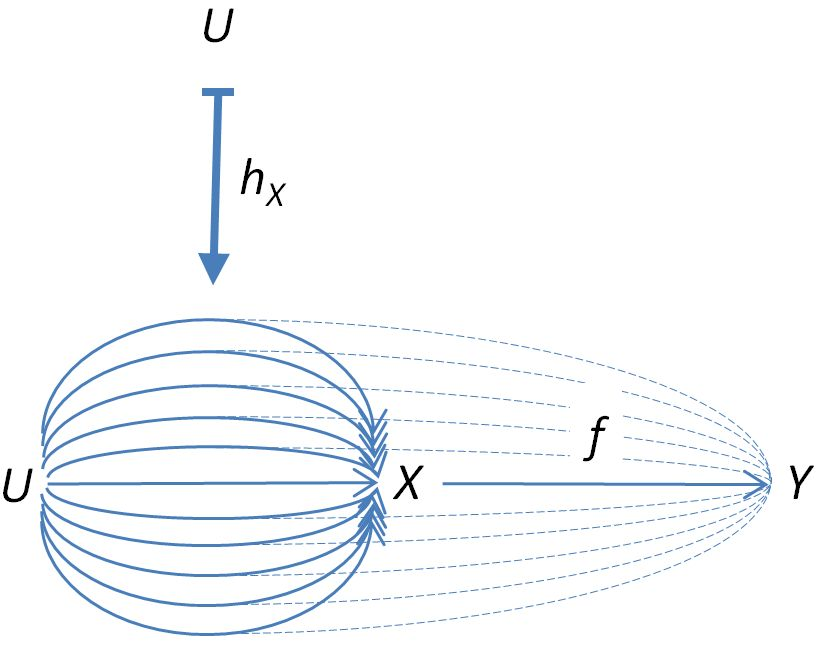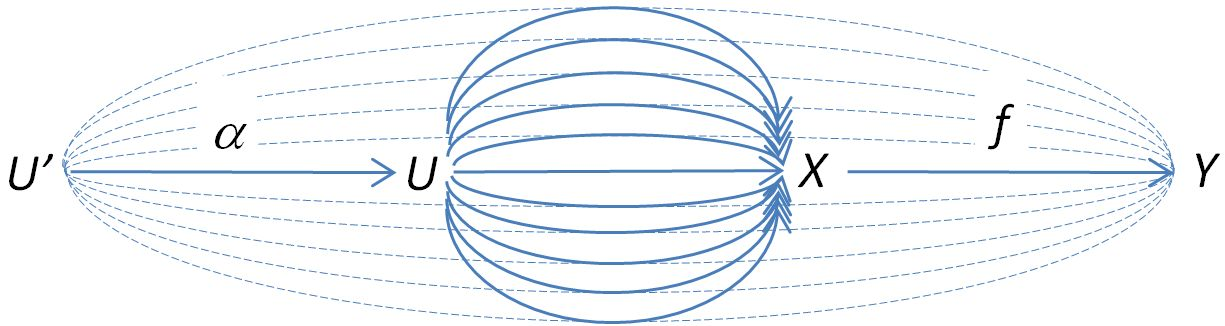Contents

category theory

Yoneda lemma

# Contents

## Idea

For $C$ a locally small category, every object $X$ of $C$ induces a presheaf on $C$: the representable presheaf $h_X$ represented by $X$. This assignment extends to a functor $C \to [C^{op}, Set]$ from $C$ to its category of presheaves. The Yoneda lemma implies that this functor is full and faithful and hence realizes $C$ as a full subcategory inside its category of presheaves.

Recall from the discussion at representable presheaf that the presheaf represented by an object $X$ of $C$ is the functor $h_X :C^{op} \to Set$ whose assignment is illustrated bywhich sends each object $U$ to $Hom_C(U,X)$ and each morphism $\alpha:U'\to U$ to the function

$h_X\alpha: Hom_C(U,X)\to Hom_C(U',X).$

Moreover, for $f : X \to Y$ a morphism in $C$, this induces a natural transformation $h_f : h_X \to h_Y$, whose component on $U$ in $X$ is illustrated byFor this to be a natural transformation, we need to have the commuting diagram

$\array{ h_X U & \stackrel{h_f U}{\rightarrow} & h_Y U \\ \mathllap{h_X\alpha\quad}{\downarrow} & {} & \mathrlap{\downarrow}{\quad h_Y\alpha} \\ h_X U' & \stackrel{h_f U'}{\rightarrow} & h_Y U' }$

but this simply means that it doesn’t matter if we first “comb” the strands back to $U'$ and then comb the strands forward to $Y$, or comb the strands forward to $Y$ first and then comb the strands back to $U'$which follows from associativity of composition of morphisms in $C$.

## Definition

The Yoneda embedding for $C$ a locally small category is the functor

$Y : C \to [C^{op}, Set]$

from $C$ to the category of presheaves over $C$ which is the image of the hom-functor

$Hom : C^{op} \times C \to Set$

$Hom(C^{op} \times C , Set) \simeq Hom(C, [C^{op}, Set])$

Hence $Y$ sends any object $c \in C$ to the representable presheaf which assigns to any other object $d$ of $C$ the hom-set of morphisms from $d$ into $c$:

$Y(c) \;\colon\; C^{op} \stackrel{C(-,c)}{\to} Set \,.$

The Yoneda embedding is sometimes denoted by よ, the hiragana kana for “Yo”; see the references below.

## Remarks

We can also curry the Hom functor in the other variable, thus obtaining a contravariant functor

$C^{op} \to [C, Set]$

which is explicitly given by $c \mapsto C(c,-)$. This is sometimes jokingly called the contravariant Yoneda embedding.

However, since $C^{op}(-,c)=C(c,-)$, it is easy to see that the contravariant Yoneda embedding is just the Yoneda embedding $Y: C^{\op} \to [(C^{op})^{op}, Set]=[C, Set]$ of $C^{op}$, and hence does not require special treatment.

## Properties

###### Proposition

(Yoneda embedding is a fully faithful functor)

For $\mathcal{C}$ any category, the functor

$\array{ \mathcal{C} &\overset{Y}{\hookrightarrow}& [\mathcal{C}^{op}, Set] \\ c &\mapsto& Hom_{\mathcal{C}}(-,c) }$
###### Proof

We need to show that for $c_1, c_2 \in \mathcal{C}$ any two objects, we have that every morphism of presheaves between their represented presheaves

$Hom_{\mathcal{C}}(-,c_1) \overset{\phi}{\longrightarrow} Hom_{\mathcal{C}}(-,c_2)$

is of the form

$\phi \;=\; Hom_{\mathcal{C}}(-,f)$

for a unique morphism

$f \;\colon\; c_1 \to c_2$

in $\mathcal{C}$. This follows by the Yoneda lemma, which says that morphisms $\phi$ as above are identified with the elements in

$Hom_{\mathcal{C}}(-,c_2)(c_1) \;=\; Hom_{\mathcal{C}}(c_1,c_2) \,.$

It is also limit preserving (= continuous functor), but does in general not preserve colimits.

The Yoneda embedding of a small category $S$ into the category of presheaves on $S$ gives a free cocompletion of $S$.

If the Yoneda embedding of a category has a left adjoint, then that category is called a total category .

### General

Early accounts:

• Alexander Grothendieck, Section A.1 of: Technique de descente et théorèmes d’existence en géométrie algébriques. II. Le théorème d’existence en théorie formelle des modules, Séminaire Bourbaki : années 1958/59 - 1959/60, exposés 169-204, Séminaire Bourbaki, no. 5 (1960), Exposé no. 195, 22 p. (numdam:SB_1958-1960__5__369_0)

For more see at Yoneda lemma the list of references given there.

### Notation

It seems that the notation “よ” for the Yoneda embedding (the hiragana kana for “Yo”) was first used in

• Theo Johnson-Freyd, Claudia Scheimbauer, p. 33 of (Op)lax natural transformations, twisted quantum field theories, and “even higher” Morita categories, (arxiv:1502.06526)

Their Latex code for the command reads as follows:

\usepackage[utf8]{inputenc}

\DeclareFontFamily{U}{min}{}

\DeclareFontShape{U}{min}{m}{n}{<-> udmj30}{}

\newcommand\yo{\!\text{\usefont{U}{min}{m}{n}\symbol{'207}}\!}

Subsequent references that use this notation include: